# Field (mathematics) facts for kids

Kids Encyclopedia Facts

In mathematics, a field is a certain kind of algebraic structure. In a field, one can add (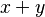$x+y$), subtract (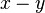$x-y$), multiply (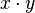$x\cdot y$) and divide (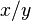$x/y$) two numbers (with division only possible if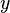$y$ is non-zero). A field is a special ring in which division is possible. Both the set of rational numbers and the set of real numbers are examples of fields. One can also use the symbol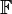$\mathbb{F}$ as a variable for a field.

## Rules

Since a field is always a ring, it consists of a set (represented here with the letter R) with two operations: addition (+) and multiplication (•). The properties of a ring are:

• Closure: If an operation is used on any elements in the ring, the element that is formed will also be part of the ring.
• For all a, b in R, the result of the operation a + b is also in R.
• For all a, b in R, the result of the operation a • b is also in R.
• Additive Identity element: One element of the ring is special. It is called the additive identity element named zero. If we add zero to any element, that element will not change.
• There exists an element 0 in R, such that for all elements a in R, the equation 0 + a = a + 0 = a holds.
• Associativity of Addition: When addition is done many times, it does not matter how it is grouped, the result will be the same.
• For all a, b and c in R, the equation (a + b) + c = a + (b + c) holds.
• Commutativity of addition: When addition is done, it does not matter which element is on the right and left, the result will be the same.
• For all a and b in R, the equation a + b = b + a holds.
• Additive Inverse element: Every element in the ring has another element in the ring, such that when addition is performed between them, the result is zero. This is known as its additive inverse.
• For each a in R, there exists an element -a in R such that a + (-a) = 0, where 0 is the additive identity element.
• Associativity of multiplication: When multiplication is done many times, it does not matter how it is grouped, the result will be the same.
• For all a, b and c in R, the equation (a • b) • c = a • (b • c) holds.
• Distribution: Addition and multiplying mix together nicely:
• For all a, b and c in R, the equation (a + b) • c = (a • c) + (b • c) holds.
• For all a, b and c in R, the equation a • (b + c) = (a • b) + (a • c) holds.

A field is a ring (so it follows the rules above), with these extra rules:

• Commutativity of multiplication: When multiplication is done, it does not matter which element is on the right and left, the result will be the same.
• For all a and b in R, the equation a • b = b • a holds.
• Multiplicative Identity element: One element of the ring is special. It is called the multiplicative identity element named one, and is different to zero. If we multiply one with any element, that element will not change.
• There exists an element 1 (different to 0) in R, such that for all elements a in R, the equation 1 • a = a • 1 = a holds.
• Multiplicative Inverse element: Every element in the ring apart from the zero element has an inverse. Multiplying an element by its inverse will give one.
• For each a in R, apart from 0, there exists an element a-1 such that a • a-1 = a-1 • a = 1 holds.

Another way of describing a field is this:

• The set R using the operation + is an abelian (commutative) group with the zero element as its identity element.
• The set R, without the zero element, using the operation • is also an abelian group, with the unit element as its identity element.
• The field also satisfies the distribution rules, listed above.

## Examples of fields

Examples of fields include:

• The set of rational numbers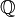$\mathbb{Q}$
• The set of real numbers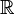$\mathbb{R}$
• The set of complex numbers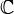$\mathbb{C}$

In fact, among the subsets of complex numbers, the largest field is$\mathbb{C}$ and the smallest field is$\mathbb{Q}$. The set of integers,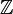$\mathbb{Z}$, is not a field, because the result of a division is not always an integer.

## Images for kidsField (mathematics) Facts for Kids. Kiddle Encyclopedia.